# NUMBERVALUE Function

Converts a text string into a number in a locale-independent manner

## What is the NUMBERVALUE Function?

The NUMBERVALUE Function is categorized under Excel TEXT functions. It will convert a text string into a number in a locale-independent manner.

The function was added in MS Excel 2013 and not available in earlier versions.

### Formula

=NUMBERVALUE(Text,[decimal_separator],[group_separator])

The NUMBERVALUE function uses the following arguments:

1. Text (required argument) – This is the text that will be converted into a number.
2. Decimal_separator (optional argument) – The character used to separate the integer and fractional part of the result.
3. Group_separator (optional argument) – Is used to separate groupings of numbers, such as separating millions from thousands, thousands from hundreds, etc.

### How to use the NUMBERVALUE Function in Excel?

Let’s see a few examples to understand how the NUMBERVALUE function works:

#### Example 1

Suppose we input the formula NUMBERVALUE(“1.500,7″,”,”,”.”)), the function will return 1500.70. Here, the decimal separator of the text argument in the example is specified in the second argument as a comma, and the group separator is specified in the third argument as a period.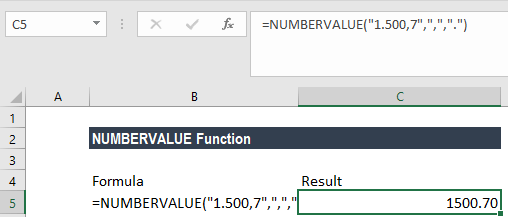Now let’s take a second example. When we input the formula ‘=NUMBERVALUE(“10%”), the function will return 0.10. This is so as no optional arguments are specified and, hence, Excel took the current locale. The function calculated the percentage, although the symbol is not shown.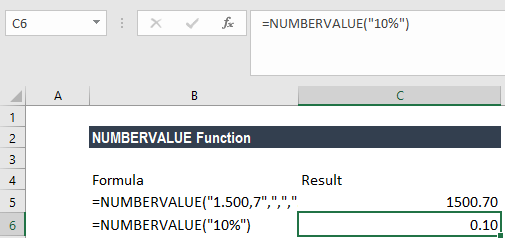#### Example 2

When the text argument ends in one or more than one percent signs (%), they will be used in the calculation of the result.

Let’s take an example. If we give the following formulas, the results will vary, as shown below: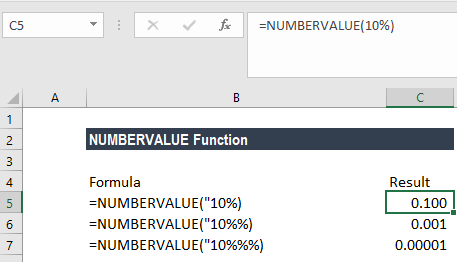As seen above, when the text argument included multiple percent signs, Excel considered each sign and produced the results accordingly. It returned the same result as the formula =10%% would.

#### Example 3

Now let’s see how we can use the NUMBERVALUE function along with other Excel functions. Suppose there is a column representing a few numbers. The numbers are in centimeters so “cm” is written at the end of the number.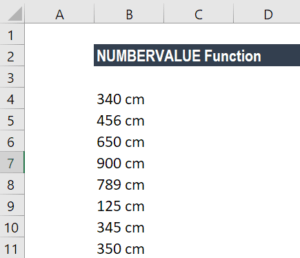If we wish to get the average of the numbers, we won’t be able to do so by simply using the AVERAGE formula as “cms” is written in the cells. To get the average, we can use the formula: =AVERAGE(NUMBERVALUE(LEFT(B4:B11,3))).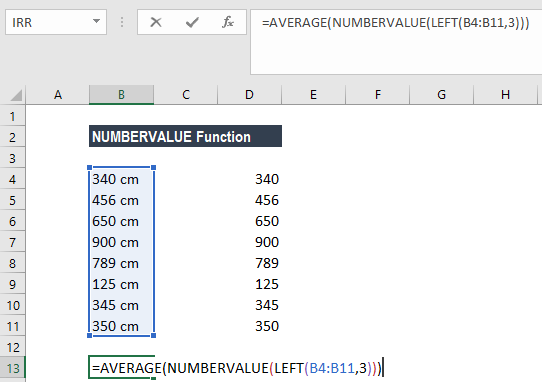If we simply press the Enter key, it will give us an error. We need to convert the original formula into an array formula. For this, we need to put in the formula in the cell and then press Ctrl-Shift-Enter to get the desired average. We can specify the number of decimals required using the decimal separator.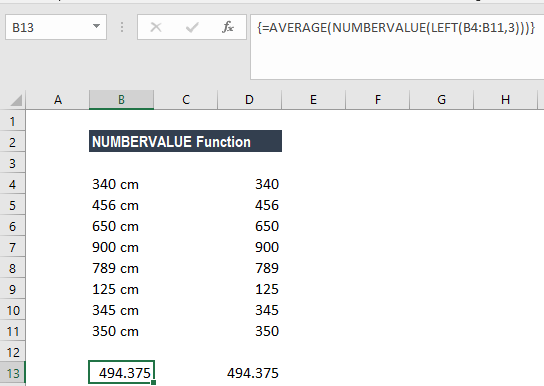In column D, we used the same numbers and showed the AVERAGE result to check the accuracy of the original formula.

### Notes about the NUMBERVALUE Function

1. When the decimal_separator and group_separator arguments are not specified, the function will use the separators from the current locale.
2. We will get the result of 0 if we specify an empty string (“”) as the text argument.
3. #VALUE! error – Occurs in the following situations:
• When the group separator occurs after the decimal separator in the text argument.
• If the given arguments are invalid.
• When decimal_separator is used more than once.
4. If there are any empty spaces in the text argument, they will be ignored. For example, ” 30 0 0 ” is returned as 3000.
5. If decimal_separator or group_separator contains more than one character, only the first character is used.
6. #NUM error – Occurs when the group_separator occurs after the decimal separator.
7. When the group_separator occurs before the decimal separator, the group separator is ignored by the function.

Thanks for reading CFI’s guide to the Excel NUMBERVALUE function. By taking the time to learn and master Excel functions, you’ll significantly speed up your financial analysis. To learn more, check out these additional CFI resources:

• Excel Functions for Finance